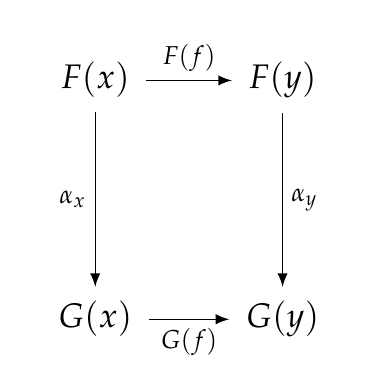Jonathan wrote:

> John wrote:
> > Maybe someone can expound a bit more on the notion of 'consistency' that I'm vaguely alluding to. All I mean is that a bunch of squares commute... but it's good to try to tease out the meaning of this, and express it in something resembling plain English.

> Well, I'm not sure if this is exactly what you're referring to, but maybe it's close?

> Generally speaking, homomorphisms identify a substructure of a target object that resembles (i.e. preserves the behavior of) the source object [...]

Your answer is great, but I was fishing for a more down-to-earth answer connected to the database examples we're looking at. Something like this:

When we change databases using a natural transformation, relationships are preserved.
For example if Vanessa is a friend of Bodo and we rename Bodo as "Herr Schmidt" and rename Vanessa as "Signora Cucinelli", naturality demands that Signora Cucinelli be a friend of Herr Schmidt!

We say a transformation \$$\alpha: F \Rightarrow G \$$ between database instances \$$F,G : \mathcal{C} \to \mathbf{Set}\$$ is **natural** if for each morphism \$$f : x \to y\$$ in \$$\mathcal{C}\$$, this square commutes:or in other words,

$G(f) \alpha_x = \alpha_y F(f) .$

In our database examples, \$$F\$$ and \$$G\$$ each map every "data type" (=object of \$$\mathcal{C}\$$ to a set of entities of that type, and every "relationship" (=morphism of \$$\mathcal{C}\$$ to a function between sets. Here I'm using the word "relationship" for morphisms of \$$\mathcal{C}\$$ simply because we've seen examples like

$\textrm{FriendOf} : \textrm{German} \to \textrm{Italian} .$

The natural transformation \$$\alpha\$$ "renames" elements of the set \$$F(x)\$$, turning them into elements of \$$G(x)\$$ via

$\alpha_x : F(x) \to G(x) .$

Similarly, it renames elements of \$$F(y)\$$, turning them into elements of \$$G(y)\$$. We want this renaming process to be **consistent** in the sense that if \$$a \in F(x) \$$ is related to \$$b \in F(y)\$$ by the relationship \$$f\$$ in our first database:

$b = F(f) (a)$

then \$$\alpha_x (a) \in G(x) \$$ is related to \$$\alpha_y (b) \in G(y)\$$ by the relationship \$$f\$$ in our second database:

$\alpha_y (b) = G(f) (\alpha_x(a)) .$

So for example if \$$b = \$$ Vanessa is a friend of \$$a = \$$ Bodo and we rename Bodo as "Herr Schmidt" and rename Vanessa as "Signora Cucinelli", we want Signora Cucinelli to be a friend of Herr Schmidt.

Saying

$b = F(f) (a) \quad \implies \quad \alpha_y (b) = G(f) (\alpha_x(a))$

is just another way of saying that this square commutes: